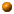# Energy CalculationsPower delivered by the hot water heater at a flow of 3 gal/min:
```(130-50)deltaF; shv_water; 3 gal/min ? kilowatt
= 35.2195 kilowatt
```

On page 36 of the September 93 issue of Popular Science there is an article titled "Green turbines" in which it is stated that a 30 megawatt wood burning electrical generation plant requires about 27000 acres of forest, but could run indefinitely by selective logging. Lets consider this statistic in other terms for comparison:First of all, how big is a 27000 acre forest?

```sqrt(27000 acre) ? miles
= 6.49519 miles  (as a square area)
```At a conversion efficiency of 40%, how much wood would be required on a daily basis? (Calchemy knows that the heat of combustion for wood by mass is approximately 8750 btu/lbm, pretty much independent of the type of wood)
```30 megawatt / (hcm_wood * 40%) ? tons/day
= 350.962 tons/day
```What's that in tons per acre per year?
```30 megawatt ; hcm_wood * 40% ; 27000 acre? ton / acre / year
= 4.74448 ton / acre / year
```Or in cords of pine per year
```(350 tons / day) / dens_pine ? cords / year
= 58135.1 cords / year
```How many trees is that? Guess that a tree ready for harvest is about 25 ft tall and about 1 foot in diameter at its base shaped as a "right circular cone" (V = 1/3 pi r^2 h).
```
(58135.1 cords / year) / ((1/3) pi (.5 ft)^2 * 25 ft/tree) ? trees/day
= 3114.92 trees / day
```About How many trees are in a 27000 acre forest? (Guess that the trees are about 10 feet apart on a more or less square grid.)
```27000 acre / ((10 ft * 10 ft) / tree) ? trees
= 1.17612E+07 trees
```About 11 million trees, so what percentage is harvested in a year?
```11 million trees ; 3114.92 trees / day ? % / year
= 10.3359 % / year
Harvesting about 10 percent per year,  each tree would have about 10 years to grow.
```Assuming an average power from the sun of about 200 watts / square meter, what is the overall solar efficiency of the system?
```30 megawatt / (27000 acres * (200 watt/square meter)) ? %
= 0.137281 %
```
The entire facility operates at a solar to electrical conversion efficiency of about 0.14 percent!How many "AA" Alkaline batteries does it take to heat a cup of coffee?

Assume 1 cup coffee = 6 oz, starts at 50 degF, ends at 212.
"E_alkaline_AA" is energy in this type of battery (fuels database in Windows version)
For Reference E_alkaline_AA = 3.21 watt hours

```shv_water; 6 floz; (212-50) deltaF ? E_alkaline_AA
= 5.78591 E_alkaline_AA
```

Calchemy Software Inc.
Fort Collins, COLast Updated 6/21/2009